# Exact Differential Equations

Many differential equations have solutions that can be written in implicit form:

F(x,y) = C

Now instead of starting with the differential equation and finding the solution, suppose we look at this backwards'': start with the solution F(x,y) = C and differentiate it, obtaining a differential equation. For example, the solutions

y2 + x y = C

would lead to the differential equation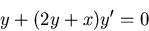In general, we have (with y = y(x))An equation of the form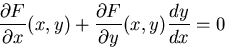is said to be an exact differential equation. Thus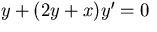is exact, and we could make as many examples as we want by taking an arbitrary (differentiable) F and differentiating.

Now let's look forwards'' again: we have a differential equation which we suspect might be exact. We need to be able to

• test whether or not is exact, and
• if so, find the function F.

Thus we want to be able to look at the differential equationand see that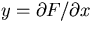and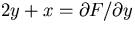where F = x y + y2, and therefore that the general solution is x y + y2 = C.

The test for exactness comes from the equality of mixed partial derivatives: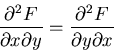So ifis exact, we must have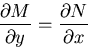In our example that is indeed the case: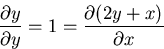It turns out that (under suitable conditions) this test is all you need. The details are as follows:

Suppose M, N,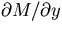and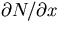are continuous in a rectangular region R: a < x < b, c < y < d. Then the differential equationis exact in R (i.e. there is a function F defined in R with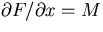and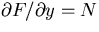there) if and only if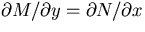everywhere in R.

Now how do we find F when the equation is exact? By integration. In our example, we wantand. Look at the first equation, and integrate with respect to x: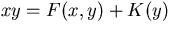A few points to notice:

• y is considered as constant when you differentiate or integrate with respect to x.
• Integratingwith respect to x gives F + a constant'', but there's no reason for this constant'' to be the same for different values of y. Therefore we have K(y) rather than just K.
Now we plug F(x,y) = x y - K(y) into the second equation.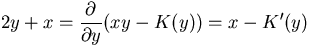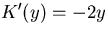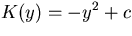This time the constant'' c really is constant, and we can ignore it (since at the end the solution F(x,y) = C will have a constant in it anyway). We conclude that F(x,y) = x y + y2 = C is the general solution of our differential equation.

Thus you can use the following procedure for solving an exact equation:

• Test that the equation really is exact: you must have.
• Integrate M with respect to x: write.
• Differentiate this with respect to y: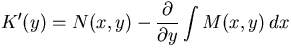. Make sure this is a function of y only, with no x in it (after whatever simplifications are necessary). If it does depend on x, you've made a mistake somewhere.
• Integrateto get K(y).
• Now we have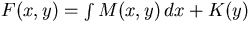, i.e. the general solution iswhere C is an arbitrary constant.

Robert Israel
1/13/2002